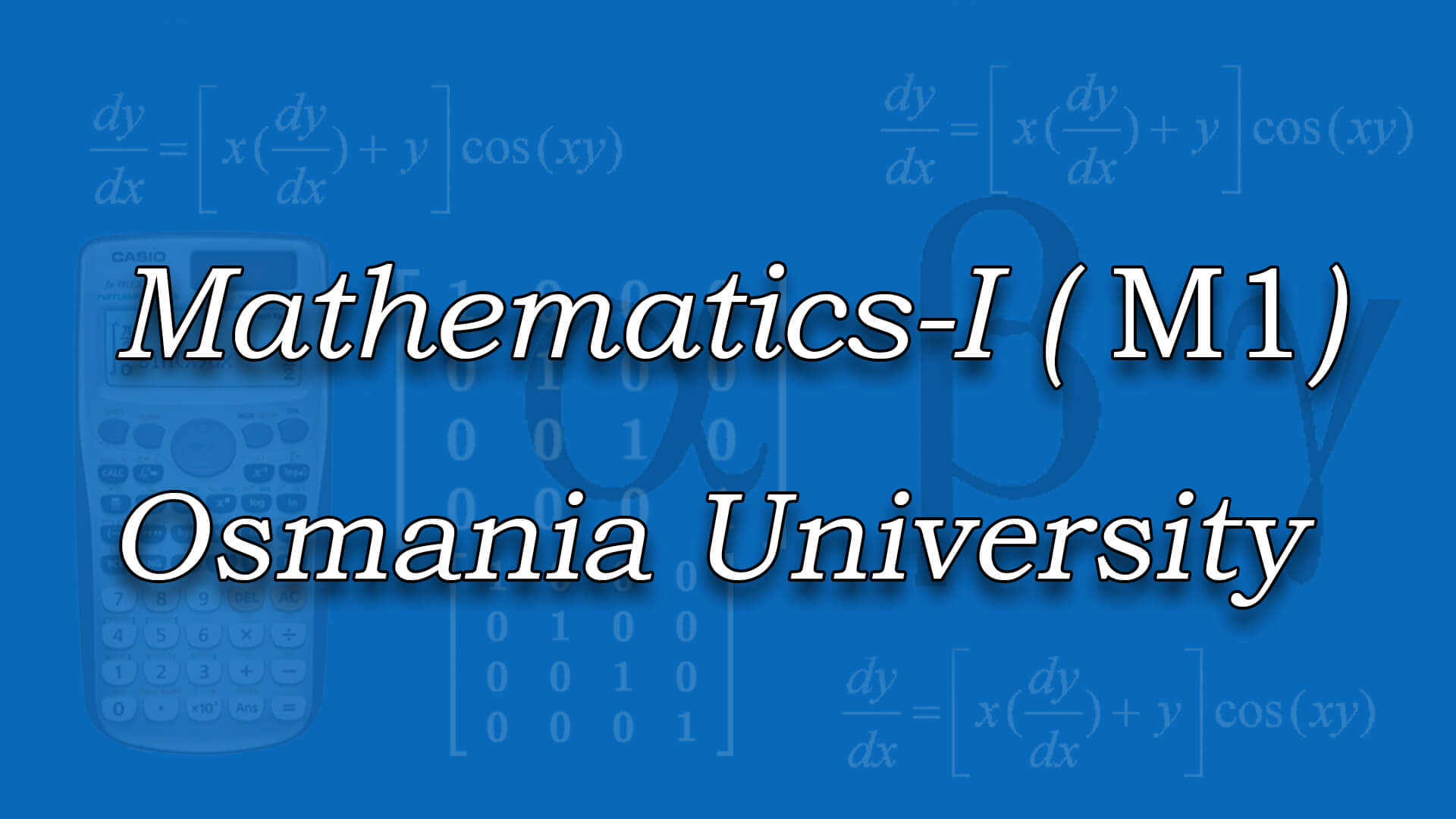## Mathematics - I for OU by Mr C Srinivas MSc ,BEd (Osmania) The course is designed to equip the students with the necessary mathematical skills and techniques that are essential for an engineering course. The skills derived from the course will help the student from a necessary base to develop analytic and design concepts. Understand the most basic numerical methods to solve simultaneous linear equations. Code : M1OU Recommended For : B.Tech 1st Year OU

#### After Learning this subject,you should be able to

• Determine rank, Eigenvalues and Eigen vectors of a given matrix and solve simultaneous linear equations.
• Solve simultaneous linear equations numerically using various matrix methods.
• Analyse the nature of sequence and series.
• Solve the applications on the mean value theorems.
• Calculate gradient of a scalar function, divergence and curl of a vector function. Determine line, surface and volume integrals.
• Apply Green, Stokes and Gauss divergence theorems to calculate line, surface and volume integrals.

## Curriculum 09:39:13   units   |   5

• 4:01:57

UNIT 1
Linear Algebra: Types of real matrices and complex matrices, Types of real matrices and complex matrices Part 1, Types of real matrices and complex matrices Part 2, Types of real matrices and complex matrices Part 3, Rank Of Matrix, Rank Of Matrix Problem, Echelonform Problem Part 1, Echelonform Problem Part 2, Solution of system of Linear Equations, Solution of system of Homogeneous Linear Equations, Consistency and solution of linear systems(Homogeneous and Non-Homogeneous) Part 1, Consistency and solution of linear systems(Homogeneous and Non-Homogeneous) Part 2, Eigen Values and Eigen Vectors, Eigen Values and Eigen Vectors and their properties Theorem, Eigen Values and Eigen Vectors and their properties Theorem Part 1, Eigen Values and Eigen Vectors Problems Part 1, Eigen Values and Eigen Vectors Problems Part 2, Eigen Values and Eigen Vectors Problems Part 3, Diagonalization of matrix, Diagonalization of a matrix by Orthogonal Reduction, Diagonalization of matrix (Problem 1), Diagonalization of matrix (Problem 2), Cayley-Hamilton Theorem(Without Proof), Cayley-Hamilton Theorem Problem, Quadratic Forms and Nature Of The Quadratic Form, Quadratic Forms and Nature of the Quadratic forms part 1, Quadratic Forms and Nature of the Quadratic forms part 2

• 1:19:16

UNIT 2
Infinite Series: Definition of Sequence,Limit,Convergent,Divergent and Oscillatory sequences, Convergent,Divergent and Oscillatory series Part 1, Convergent,Divergent and Oscillatory series Part 2, Sequences and Series, Limit Comparison Test, Limit Comparison Test Problem 1, Limit Comparison Test Problem 2, Limit Comparison Test Problem 3, P-Test & D'Alemberts Ratio Test, Raabe's test, Cauchy's nth Root Test & Cauchy's Integral Test, Cauchy's Integral test and Cauchy's root test Problem 1, Cauchy's Integral test and Cauchy's root test Problem 2, Cauchy's Integral test and Cauchy's root test Problem 3, Logarithmic test, Alternating series : Leibnitz test, Alternating series : Leibnitz test Problem, Alternating Convergent series : Absolute and Conditionally convergence

• 0:44:14

UNIT 3
Differential Calculus: Rolle's Theorem, Geometrical Interpretation Of Rolle's Theorem, Mean Value Theorems:Rolle's Theorem Part 1, Mean Value Theorems:Rolle's Theorem Part 2, Mean Value Theorems:Rolle's Theorem Part 3, Lagrange's Mean Value Theorem, Geometrical Interpretation Of Lagrange's Mean Value Theorem, Lagrange's Mean value theorem and applications Part 1, Lagrange's Mean value theorem and applications Part 2, Cauchy's Mean Value Theorem, Taylor's Series

• 1:14:12

UNIT 4
Functions of Several Variables: Limits and continuity of functions of two variables, Partial Derivatives, Total Differentials and Derivatives, Derivatives of composite and implicit functions, Higher order partial derivatives, Taylor's theorem for functions of two variables, Taylor's theorem for functions of two variables Part 1, Taylor's theorem for functions of two variables Part 2, Jacobian Determinant, Jacobian Problem 1, Jacobian Problem 2, Maxima and Minima Functions of two variables, Maxima and Minima Functions of two variables Part 1, Maxima and Minima Functions of two variables Part 2

• 2:19:34

UNIT 5
Vector Calculus: Introduction to Vector Differentiation, Gradient, Directional derivative, Problems on Gradient and Directional Derivative - Part 1, Problems on Gradient and Directional Derivative - Part 2, Divergence, Curl, Problems on Gradient,Divergence and Curl, Problems on Gradient,Divergence and Curl Part 1, Line Integral, Potential Function, Area,surface and volume of integrals, Greens Theorem, Green's Theorem Problems, Stokes Theorem, Stokes Theorem Part 1, Stokes Theorem Part 2, Gauss Divergence theorem, Gauss Divergence theorem Part 1